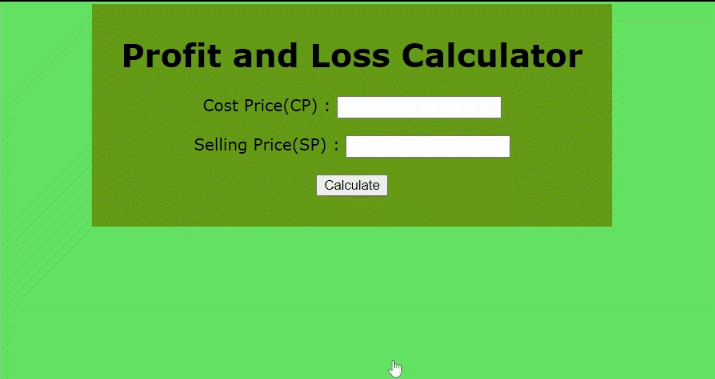Create a Profit and Loss Calculator using JavaScript

• Last Updated : 10 Oct, 2021

In this article, we will create a profit & loss calculator using HTML, CSS & Javascript for adding the basic functionality along with adding the design and layout. Profit and Loss Calculator is basically used to calculate the amount or percentage received after selling a particular price or goods. If the amount(Selling Price) received is greater after selling than the actual amount(Cost Price), it is considered profit otherwise loss. We will denote cost price as CP & selling price as SP.

Formula Used:

• Profit: (SP) – (CP)
• Profit Percentage: Profit/CP x 100
• Loss: (SP) – (CP)
• Loss Percentage: Loss/CP x 100

Approach:

• In the body tag, create the design and layout of the calculator using basic HTML.
• Use CSS properties for stylings such as alignment, size, background, etc.
• To calculate profit and loss call a function using JavaScript.

Example: We will use the above approach to create a calculator.

HTML



Profit and Loss Calculator

Cost Price(CP) :

Selling Price(SP) :

CSS Code:

CSS

 body {  background-color: rgb(99, 226, 99);  font-family: Verdana;}.plcalculate {  text-align: center;  background-color: rgb(102, 155, 22);  width: 500px;  margin-left: auto;  margin-right: auto;  padding: 10px;}h2 {  color: white;}

Javascript:

Javascript

 function Calculate() {  const CP = document.querySelector(".cost__price").value;  const SP = document.querySelector(".selling__price").value;    const profit__loss = document.querySelector(".profit__loss");  const percentage = document.querySelector(".profit__loss__percentage");  const nothing = document.querySelector(".nothing");    profit__loss.innerHTML = "";  percentage.innerHTML = "";  nothing.innerHTML = "";    if (SP > CP) {    const profit = SP - CP;    const profit_percent = ((profit / CP) * 100).toFixed(2);      profit__loss.innerHTML = "Profit : " + profit;    percentage.innerHTML = "Profit Percentage : " + profit_percent;  }  if (SP < CP) {    const loss = CP - SP;    const loss_percent = ((loss / CP) * 100).toFixed(2);      profit__loss.innerHTML = "Loss : " + loss;    percentage.innerHTML = "Loss Percentage : " + loss_percent;  }  if (SP == CP) {    nothing.innerHTML = "No Profit No Loss";  }};

Explanation: A Calculate() function will be invoked when the user enters the CP and SP amount in the input and clicks the Calculate button using the onclick event attribute. In this function, we have used DOM querySelector() method to select the value entered in the inputs into a variable using their class names. If the SP amount is greater than the CP amount then Calculate() function will calculate the profit and profit percentage otherwise calculate the loss and loss percentage using the above-mentioned formulae and displayed the text using the innerHTML property.

Complete Code:

HTML

     Profit and Loss Calculator

Profit and Loss Calculator

Cost Price(CP)   :

Selling Price(SP)   :

Output:Hey geek! The constant emerging technologies in the world of web development always keeps the excitement for this subject through the roof. But before you tackle the big projects, we suggest you start by learning the basics. Kickstart your web development journey by learning JS concepts with our JavaScript Course. Now at it's lowest price ever!

My Personal Notes arrow_drop_up